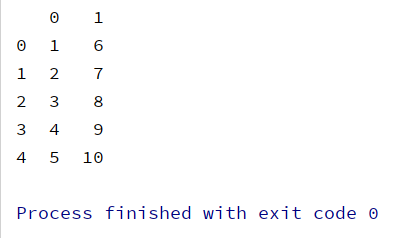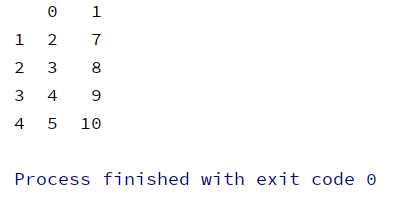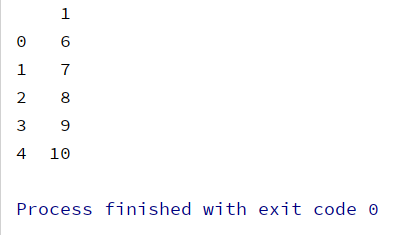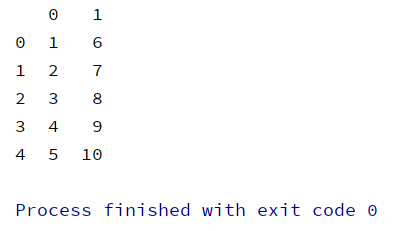pandas 中的 drop 方法是很明智的数据清理的方法，它的好处在于：它不改变原有的 df 中的数据，而是返回另一个新的 DataFrame 来存放删除后的数据。

# 一、drop 的用法

``````import pandas as pd
import numpy as np

a = list(range(1, 11))
a_reshape = np.array(a).reshape(2, 5).T
b = pd.DataFrame(a_reshape)
print(b)``````## 1. df.drop([row_num], axis=0)：删除 DataFrame 某一行（如果不指定axis，那么默认axis=0）

``````c = b.drop(0, axis=0)
print(c)``````## 2. df.drop([row_num], axis=1)：删除 DataFrame 某一列

``````d = b.drop(0, axis=1)
print(d)``````# 二、python 中数据清理的常见方法

## 1. 删除无效项

（1）df[df.isnull()] 和 df[df.notnull()]：df.isnull() 和 df.notnull() 返回的是一个 true 或 false 的 Series对象，所以这种方式可以筛选出我们需要的特定数据。

（2）df.dropna()：将所有含有 nan 项的 row 删除（默认：axis=0） 。

（3）df.dropna(axis=1, thresh=3)：将在列方向上有三个为 nan 的列删除。

（4）df.dropna(how='ALL')：将全部项都是 nan 的 row 删除。

## 2. 填充空缺项

（1）df.fillna(0)

（2）df.fillna({1:0, 2:0.5})：对第一列 nan 值赋0，第二列赋值 0.5；

（3）df.fillna(method='ffill')：在列方向上用前一个值赋值给 nan。

# Tips：

### 1. 注意：drop() 方法不改变原有的 df 数据！

``````b.drop(0, axis=1)
print(b)``````